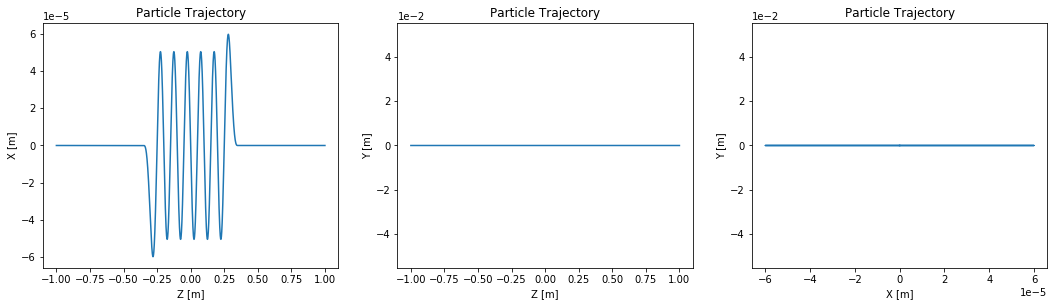# Particle Beams¶

This example describes all of the major capabilities of particle beams in OSCARS. There are pre-defined particle types (default is electron, but proton, pion, etc are included) and the user is free to describe any other particle type they wish. Non-zero beam widths can be defined using the emittance, beta functions, and lattice reference (point at which the beta functions are defined). One can also define multiple particle beams in one OSCARS object and give weights to each.

Patricle beams can as well be defined, like other OSCARS objects, in any position and direction. Although examples are often given where $+\hat z$ is the beam direction (mostly to align with accelerator nomenclature), there is no preferred direction in OSCARS.

The minimum definition for a particle beam must include calling the two functions:

• set_particle_beam()
• set_ctstartstop(tstart, tstop)

where tstart and tstop are the start and stop times for particle propoation. In the above set_particle_beam() will use all of its defaults (since no arguments are given) which is essentially an electron at rest at the origin. The defaults for set_particle_beam() are:

• type='electron'
• name (auto-assigned)
• energy_GeV=0
• x0=[0, 0, 0]
• d0=[0, 0, 1]
• t0=0
• current=1.602176462e-19 (single electron charge)
• emittance=[0, 0]

The elements which you may want to define for a beam are:

• type (one of the predefined types, or 'custom')
• name (any unique name you like)
• energy_GeV (the energy in GeV)
• x0 (position where initial conditions are given)
• d0 (direction of beam at initial position)
• current (the current in [A])

For a non-zero emittance beam you must additionally define the following (the beam shape is taken to be gaussian, though other distributions are possible):

• sigma_energy_GeV (width in energy spread in [GeV])
• emittance (the beam emittance in [m.rad] as [h, v])
• beta (the beta function in [m] as [h, v])
• lattice_reference (the reference point at which beta is defined)
• horizontal_direction (the horizontal direction of the beam)

For a particle beam you can additionally define the following:

• rotations (rotate the beam about x, then y, then z given as [$\theta_x, \theta_y, \theta_z$])
• translation (translate the beam in x, y, z given as [x, y, z])
• t0 (time at the position x0 defined above, default is 0)
• weight (for randome particle generation with multiple beams, default is 1)

Custom: If you set type='custom' you will need to also define the following:

• mass (in [kg])
• charge (in [C])

The two functions for adding a particle beam are:

• set_particle_beam() - Removes all other particle beams
• add_particle_beam() - The same as set but does not remove previous beams

To see what beam(s) are setup in OSCARS you may call:

• print_particle_beams()

or to see everything we recommend:

• print_all()
In :
# matplotlib plots inline
%matplotlib inline

# Import the OSCARS SR module
import oscars.sr

# Import OSCARS plots (matplotlib)
from oscars.plots_mpl import *

OSCARS v2.1.8 - Open Source Code for Advanced Radiation Simulation
Brookhaven National Laboratory, Upton NY, USA
http://oscars.bnl.gov
oscars@bnl.gov

In :
# Create an OSCARS SR object
osr = oscars.sr.sr()

# For these examples we will make use of a simple undulator field
osr.add_bfield_undulator(bfield=[0, 1, 0], period=[0, 0, 0.100], nperiods=5)

plot_bfield(osr)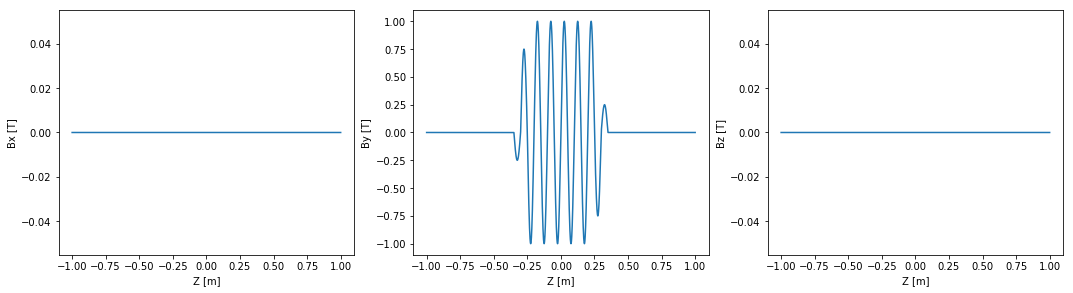## Basic beams / Particles¶

These are basic beams with zero emittance, one electron (NSLSII energy) and one proton (LHC energy)

### Electron¶

First example is showing full definition, second using the defaults for less typing.

In :
# Add a basic electron beam with zero emittance
osr.set_particle_beam(type='electron', name='beam_0', energy_GeV=3, x0=[0, 0, -1], d0=[0, 0, 1], current=0.500)

# You MUST set the start and stop time for the calculation
osr.set_ctstartstop(0, 2)

# Plot trajectory of beam
plot_trajectory_position(osr.calculate_trajectory())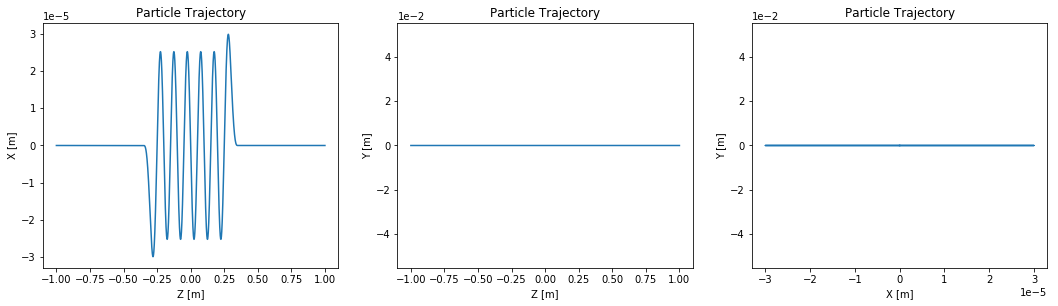In :
# Add a basic electron beam with zero emittance
osr.set_particle_beam(energy_GeV=3, x0=[0, 0, -1], current=0.500)

# You MUST set the start and stop time for the calculation
osr.set_ctstartstop(0, 2)

# Plot trajectory of beam
plot_trajectory_position(osr.calculate_trajectory())### Proton beam¶

This example shows how to define a proton beam at 13 TeV

In :
# Add a basic proton beam with zero emittance
osr.set_particle_beam(type='proton', energy_GeV=13000, x0=[0, 0, -1], current=0.500)

# You MUST set the start and stop time for the calculation
osr.set_ctstartstop(0, 2)

# Plot trajectory of beam
plot_trajectory_position(osr.calculate_trajectory())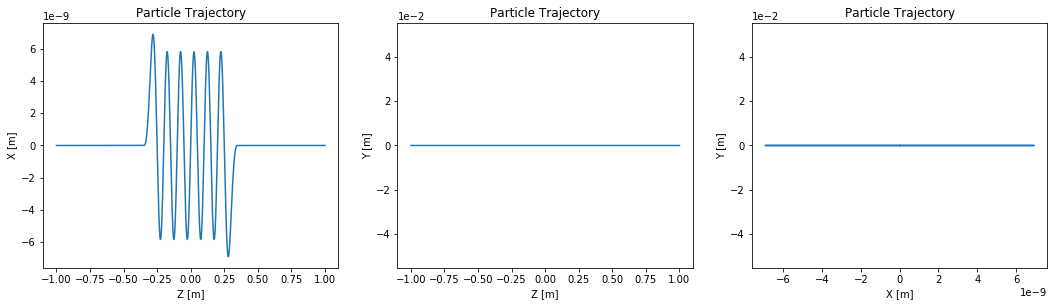## Rotating Beams¶

Beams can easily be rotated using the rotations variable. This essentialyl rotates the initial conditions given. It rotates the initial position x0 in space and rotates the direction vector d0.

In :
# Add a basic proton beam and rotate it 45 degrees
osr.set_particle_beam(type='proton',
energy_GeV=13000,
x0=[0, 0, -1],
current=0.500,
rotations=[osr.pi()/4., 0, 0]
)

# You MUST set the start and stop time for the calculation
osr.set_ctstartstop(0, 2)

# Plot trajectory of beam
plot_trajectory_position(osr.calculate_trajectory())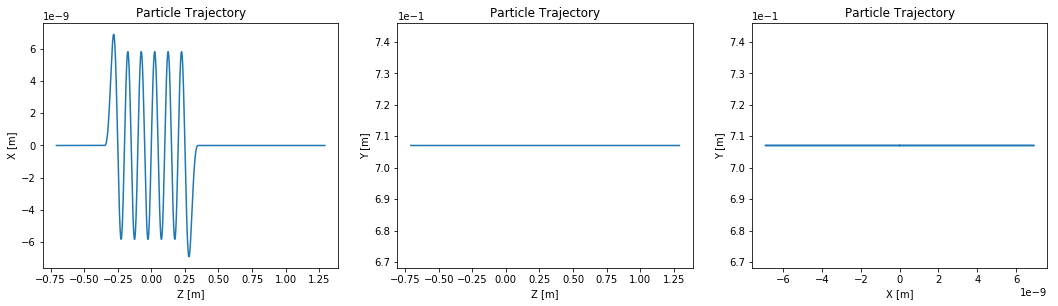## Multiple beams¶

This examples defined multiple beams in the same object, then plots the trajectory for two random particles selected from these beams.

Instead of using set_particle_beam we will use add_particle_beam (which is really the same thing, but set_particle_beam issues a clear_particle_beams() internally)

In :
# Add basic electron beams with zero emittance
osr.clear_particle_beams()
osr.add_particle_beam(type='electron', name='beam_0', energy_GeV=3,  x0=[0, 0, -1], current=0.500)
osr.add_particle_beam(type='positron', name='beam_1', energy_GeV=3,  x0=[0, 0, -1], current=0.500)
osr.add_particle_beam(type='proton',   name='beam_2', energy_GeV=13, x0=[0, 0, -1], current=0.500)
osr.add_particle_beam(type='muon',     name='beam_3', energy_GeV=2,  x0=[0, 0, -1], current=0.500)
osr.add_particle_beam(type='pi+',      name='beam_4', energy_GeV=1,  x0=[0, 0, -1], current=0.500)

# You MUST set the start and stop time for the calculation
osr.set_ctstartstop(0, 2)

# Plot trajectory of beam
plot_trajectory_position(osr.calculate_trajectory())
osr.set_new_particle()
plot_trajectory_position(osr.calculate_trajectory())
osr.set_new_particle()
plot_trajectory_position(osr.calculate_trajectory())

# Print particle beam info for checking
osr.print_particle_beams()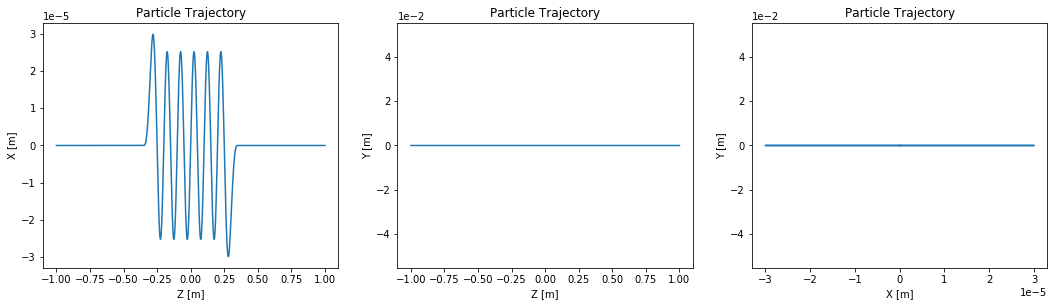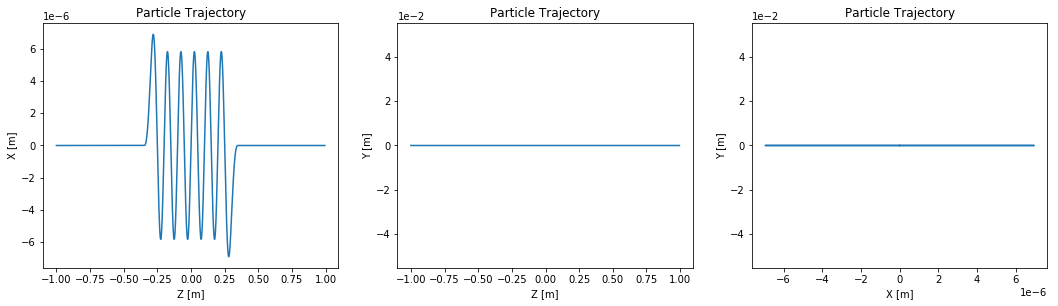TParticleBeamContainer has 5 beams
Name:             beam_0
Weight:           1
X0:               (0, 0, -1)
U0:               (0, 0, 1)
T0:               0 [m]  0 [s]
E0:               3
SigmaE:           6.95324e-310
Current           0.5
Emittance         (6.95031e-310, 6.95032e-310)
V-direction       (-0, 1, -0)
H-direction       (1, -0, -0)
BeamDistribution  filament
TwissBeta         (6.95024e-310, 6.95032e-310)
TwissAlpha        (6.95031e-310, 6.95024e-310)
TwissGamma        (4.94066e-324, 6.95032e-310)
Twiss Lattice Ref (0, 0, 0)
TwissBetaX0       (2.08509e-309, 2.78011e-309)
TwissAlphaX0      (6.95031e-310, 1.39006e-309)
TwissGammaX0      (4.94066e-324, 6.95032e-310)
Eta               (0, 0)

Name:             beam_1
Weight:           1
X0:               (0, 0, -1)
U0:               (0, 0, 1)
T0:               0 [m]  0 [s]
E0:               3
SigmaE:           6.95324e-310
Current           0.5
Emittance         (0, 6.95032e-310)
V-direction       (-0, 1, -0)
H-direction       (1, -0, -0)
BeamDistribution  filament
TwissBeta         (0, -1)
TwissAlpha        (0, 0)
TwissGamma        (1, 0)
Twiss Lattice Ref (0, 0, 0)
TwissBetaX0       (1, -1)
TwissAlphaX0      (1, 0)
TwissGammaX0      (1, 0)
Eta               (0, 0)

Name:             beam_2
Weight:           1
X0:               (0, 0, -1)
U0:               (0, 0, 1)
T0:               0 [m]  0 [s]
E0:               13
SigmaE:           6.95324e-310
Current           0.5
Emittance         (0, 6.95032e-310)
V-direction       (-0, 1, -0)
H-direction       (1, -0, -0)
BeamDistribution  filament
TwissBeta         (0, -1)
TwissAlpha        (0, 0)
TwissGamma        (1, 0)
Twiss Lattice Ref (0, 0, 0)
TwissBetaX0       (1, -1)
TwissAlphaX0      (1, 0)
TwissGammaX0      (1, 0)
Eta               (0, 0)

Name:             beam_3
Weight:           1
X0:               (0, 0, -1)
U0:               (0, 0, 1)
T0:               0 [m]  0 [s]
E0:               2
SigmaE:           6.95324e-310
Current           0.5
Emittance         (0, 6.95032e-310)
V-direction       (-0, 1, -0)
H-direction       (1, -0, -0)
BeamDistribution  filament
TwissBeta         (0, -1)
TwissAlpha        (0, 0)
TwissGamma        (1, 0)
Twiss Lattice Ref (0, 0, 0)
TwissBetaX0       (1, -1)
TwissAlphaX0      (1, 0)
TwissGammaX0      (1, 0)
Eta               (0, 0)

Name:             beam_4
Weight:           1
X0:               (0, 0, -1)
U0:               (0, 0, 1)
T0:               0 [m]  0 [s]
E0:               1
SigmaE:           6.95324e-310
Current           0.5
Emittance         (0, 6.95032e-310)
V-direction       (-0, 1, -0)
H-direction       (1, -0, -0)
BeamDistribution  filament
TwissBeta         (0, -1)
TwissAlpha        (0, 0)
TwissGamma        (1, 0)
Twiss Lattice Ref (0, 0, 0)
TwissBetaX0       (1, -1)
TwissAlphaX0      (1, 0)
TwissGammaX0      (1, 0)
Eta               (0, 0)



# Beam with non-zero emittance¶

Below is an example of a beam with non-zero memittance. We use the emittance and the beta function. You must give the reference point for the beta function definition.

In :
# Add a basic electron beam with zero emittance
osr.set_particle_beam(
energy_GeV=3,
x0=[0, 0, -1],
current=0.500,
sigma_energy_GeV=0.001*3,
beta=[1.5, 0.8],
emittance=[0.9e-9, 0.008e-9]
)

# You MUST set the start and stop time for the calculation
osr.set_ctstartstop(0, 2)

# Plot trajectory of beam
osr.set_new_particle()
plot_trajectory_position(osr.calculate_trajectory())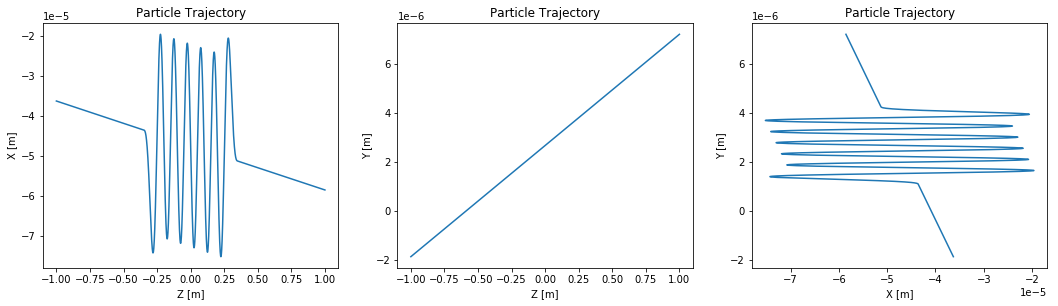In :
# Let's plot the distribution of particles at the initial position 'position'
energy = []
x = []
y = []
bx = []
by = []
for i in range(5000):
osr.set_new_particle()
energy.append(osr.get_particle_e0())
position = osr.get_particle_x0()
beta = osr.get_particle_beta0()

x.append(position)
y.append(position)

bx.append(beta)
by.append(beta)

plt.figure(1, figsize=[15, 3])

plt.subplot(131)
plt.scatter(x, y)
plt.xlim(-0.0005, 0.0005)
plt.ylim(-0.00005, 0.00005)
plt.xlabel('x0 [m]')
plt.ylabel('y0 [m]')
plt.ticklabel_format(style='sci', axis='x', scilimits=(0,0))
plt.ticklabel_format(style='sci', axis='y', scilimits=(0,0))

plt.subplot(132)
plt.scatter(bx, by)
plt.xlim(-0.0002, 0.0002)
plt.ylim(-0.00002, 0.00002)
plt.xlabel('x0 [m]')
plt.ylabel('y0 [m]')
plt.ticklabel_format(style='sci', axis='x', scilimits=(0,0))
plt.ticklabel_format(style='sci', axis='y', scilimits=(0,0))

plt.subplot(133)
plt.hist(energy)
plt.xlabel('Energy [GeV]')
plt.ticklabel_format(style='sci', axis='x', scilimits=(0,0))
plt.ticklabel_format(style='sci', axis='y', scilimits=(0,0))

plt.show()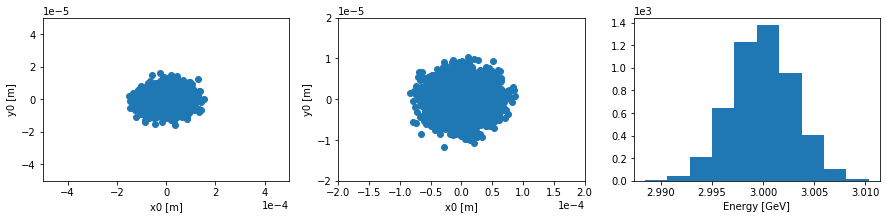## Multiple beams with non-zero emittance¶

A simple example of 2 beams with different beam parameters in the same object

In :
# Add basic electron beams with zero emittance
osr.clear_particle_beams()
energy_GeV=3,
x0=[0, 0, -1],
current=0.500,
sigma_energy_GeV=0.001*3,
beta=[1.5, 0.8],
emittance=[0.9e-9, 0.008e-9],
weight=0.7
)

energy_GeV=3.02,
x0=[0, 0.000035, -1],
d0=[0, 0, 1],
current=0.500,
sigma_energy_GeV=0.001*3,
beta=[1.5, 0.8],
emittance=[0.9e-9, 0.008e-9],
weight=0.3
)

# You MUST set the start and stop time for the calculation
osr.set_ctstartstop(0, 2)

# And plot the results
energy = []
x = []
y = []
for i in range(5000):
osr.set_new_particle()
energy.append(osr.get_particle_e0())
position = osr.get_particle_x0()

x.append(position)
y.append(position)

plt.figure(1, figsize=[10, 3])
plt.subplot(121)
plt.scatter(x, y)
plt.xlim(-0.0005, 0.0005)
plt.ylim(-0.00005, 0.00005)
plt.xlabel('x0 [m]')
plt.ylabel('y0 [m]')

plt.subplot(122)
plt.hist(energy)
plt.xlabel('Energy [GeV]')
plt.show()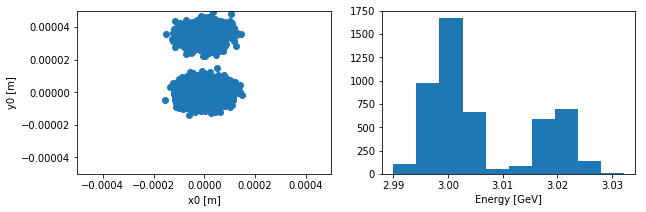## Custom Particles¶

This is an example of a custom particle in OSCARS. All that additionally needs definition are the charge and mass

In :
# Add a custom beam with zero emittance.
# This is for a particle with twice the chargs as the electron, but the same mass
osr.set_particle_beam(
type='custom',
charge=-2*osr.qe(),
mass=osr.me(),
energy_GeV=3,
x0=[0, 0, -1],
current=0.500
)

# You MUST set the start and stop time for the calculation
osr.set_ctstartstop(0, 2)

# Plot trajectory of beam
plot_trajectory_position(osr.calculate_trajectory())